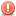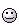A Flash Developer Resource Site

# Thread: How to get Random Array Interger???

1. ##How to get Random Array Interger???

Hi I need a way for getting random colour from an Array of hexadecimal values:

Code:
```var arr:Array = [0xFF0000,0xFF6600,0xFFFF00,0x0066FF,0x00FF00];
var colour:Number = //Random Value from arr```

Any ideas?Reply With Quote

2. PHP Code:
``` var color:uint = arr[Math.round(Math.random()*arr.length-1)];  ```Reply With Quote

3. ThanksReply With Quote

4. @ MyFriendIsATaco: your code is almost correct, but there's a tiny problem with it... By using Math.round you might end up with a situation when instead of getting a random index from 0 to the length - 1 you'll get an index of -1 and it will return undefined...

A better solution would be to use Math.ceil instead of random:

PHP Code:
``` var arr:Array = [0xFF0000, 0xFF6600, 0xFFFF00, 0x0066FF, 0x00FF00];var randIndex:Number = Math.ceil(Math.random() * arr.length - 1);var randColor:uint = arr[randIndex];  ```Reply With Quote

5. OR, I should have just used another set of parenthesis (Damn order of operations):
PHP Code:
``` var color:uint = arr[Math.round(Math.random()*(arr.length-1))];  ```
Math.ceil() is going to cause the results to NEVER be 0. It'll essentially return 1- arr.lengthReply With Quote

6. Why would ceil screw the randomness ? Please explain...

EDIT: it's essentially the same thing as using Round ( in your last post ) just that it has a slightly larger chance to hit a bigger index value than a lower one ( but that chance isn't really a significant impact ). I don't see any "scientific" reason why it would screw up the randomness...Reply With Quote

7. Hmm, you're right. But in a different way than I expected. Take a look:
PHP Code:
``` var results:Array = new Array(10);for(var i:int=0; i<10; i++) results[i] = 0;for(i=0; i<30000; i++) results[Math.ceil(Math.random()*10)-1]++;trace(results); //3059,3025,3068,2947,3076,2904,2955,3000,3010,2956  ```
Using Math.round() clumps the results in the middle. There are half as many 0s and the max as there should be:
PHP Code:
``` var results:Array = new Array(10);for(var i:int=0; i<10; i++) results[i] = 0;for(i=0; i<30000; i++) results[Math.round(Math.random()*9)]++;trace(results); //1607,3235,3466,3342,3258,3354,3326,3337,3330,1745  ```
Not quite sure why it's like that.

So I guess the best random solution to your problem would be:
PHP Code:
``` var color:uint = arr[Math.ceil(Math.random()*arr.length)-1];  ```
Notice the -1 outside the parenthesis. If it was inside, your results will always be 1 too high. 0 would never be an answer.

Any idea why Math.ceil() provides a better deviation as opposed to Math.round()?Reply With Quote

8. You got me confused a bit... Why did you use dual random instead of round and random in the second situation ?
What were you trying to prove / test there ?Reply With Quote

9. Yes.Too early in the morning.Reply With Quote

10.Originally Posted by fx.barrett
You got me confused a bit... Why did you use dual random instead of round and random in the second situation ?
What were you trying to prove / test there ?
I edited. It was an error from copying/pasting.Reply With Quote

11. Any idea why Math.ceil() provides a better deviation as opposed to Math.round()?
I don't have a scientific explanation for this... but it might have something to do with possibility. We should take a look at the Math class an see how random works ( because random ain't really random, but it's just a "mathematical illusion" ) because it might provide the answer we are looking for. Maybe it's because of the algorithm that produces that "random value", it might favor ceil but don't forget that we didn't test floor...

Or, it may have been just blind luck...Reply With Quote

12. PHP Code:
``` var results:Array = new Array(10);for(var i:int=0; i<10; i++) results[i] = 0;for(i=0; i<30000; i++) results[Math.floor(Math.random()*10)]++;trace(results); //2993,2999,2889,2950,3069,3081,3032,2979,2985,3023  ```
Floor produces some even results as well.Originally Posted by fk.barrett
Or, it may have been just blind luck...
I'm not buying that.I ran it several times to make sure, and every time, the 0 and 9 index fell right in line with ~1600. Making it just about right in the middle of where it should be, 3000.Reply With Quote

13. Even out of 1,000,000, the results are odd.
PHP Code:
``` var results:Array = new Array(10);for(var i:int=0; i<10; i++) results[i] = 0;//done in segments to avoid Flash from freaking outfor(i=0; i<50000; i++) results[Math.round(Math.random()*9)]++;for(i=0; i<50000; i++) results[Math.round(Math.random()*9)]++;for(i=0; i<50000; i++) results[Math.round(Math.random()*9)]++;for(i=0; i<50000; i++) results[Math.round(Math.random()*9)]++;for(i=0; i<50000; i++) results[Math.round(Math.random()*9)]++;for(i=0; i<50000; i++) results[Math.round(Math.random()*9)]++;for(i=0; i<50000; i++) results[Math.round(Math.random()*9)]++;for(i=0; i<50000; i++) results[Math.round(Math.random()*9)]++;for(i=0; i<50000; i++) results[Math.round(Math.random()*9)]++;for(i=0; i<50000; i++) results[Math.round(Math.random()*9)]++;for(i=0; i<50000; i++) results[Math.round(Math.random()*9)]++;for(i=0; i<50000; i++) results[Math.round(Math.random()*9)]++;for(i=0; i<50000; i++) results[Math.round(Math.random()*9)]++;for(i=0; i<50000; i++) results[Math.round(Math.random()*9)]++;for(i=0; i<50000; i++) results[Math.round(Math.random()*9)]++;for(i=0; i<50000; i++) results[Math.round(Math.random()*9)]++;for(i=0; i<50000; i++) results[Math.round(Math.random()*9)]++;for(i=0; i<50000; i++) results[Math.round(Math.random()*9)]++;for(i=0; i<50000; i++) results[Math.round(Math.random()*9)]++;for(i=0; i<50000; i++) results[Math.round(Math.random()*9)]++;trace(results);  //55586,111564,111203,110724,111023,110699,111138,111330,111049,55684var percentages:Array = new Array(10);for(i=0; i<10; i++) percentages[i] = Math.round((results[i]/1000000)*10000)/100 + "%";trace(percentages);  //5.56%,11.16%,11.12%,11.07%,11.1%,11.07%,11.11%,11.13%,11.1%,5.57%  ```
The 0 and 9 are approx equal at ~5.6% of the results. And everything else gets an ~11.1% instead of an equal 10% spread. Something is NOT coincidental here.Reply With Quote

14. Nothing is perfectNor is ActionScript...Reply With Quote

15. Yeah, well, Math.floor produces a nice even spectrum. And for the real nit-picky, probably a bit quicker to process than the Math.ceil method and Math.round method since there is no subtraction of 1 involved. No, I don't feel like running tests on that right now.

So, in the end unrealhacker12, I guess here is what the nerds have concluded:
PHP Code:
``` var color:uint = arr[Math.floor(Math.random()*arr.length)];  ```Reply With Quote

16. If optimization is important to you, you'd be far better off saving the array length to a constant somewhere and using a hard-cast to int instead of the static call to Math.floor():

PHP Code:
``` const ARR_LEN:int = arr.length; // ... var c:uint = arr[int(Math.random() * ARR_LEN)];  ```Reply With Quote

17. Oops, completely forgot about just casting to int.Thanks!Reply With Quote

18. Uhh, really late reply... Sorry.

@ neznein9: yeah, that's a good way of doing it. An even faster would be this:

PHP Code:
``` var arr:Array = [0xFF0000, 0xFF6600, 0xFFFF00, 0x0066FF, 0x00FF00];var randIndex:Number = (Math.random() * arr.length - 1) >> 0;var randColor:uint = arr[randIndex];trace(randColor);  ```
Bitwise operations will outperform everything else.Reply With Quote

19. Holy crap - bit-shifting by zero is brilliant!! I have to go benchmark some stuff right away...Reply With Quote

####Posting Permissions

• You may not post new threads
• You may not post replies
• You may not post attachments
• You may not edit your posts
•

 » Home » Movies » Tutorials » Submissions » Board » Links » Reviews » Feedback » Gallery » Fonts » The Lounge » Sound Loops » Sound FX » About FK » Sitemap